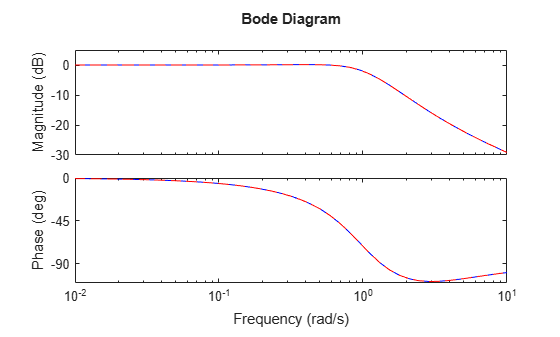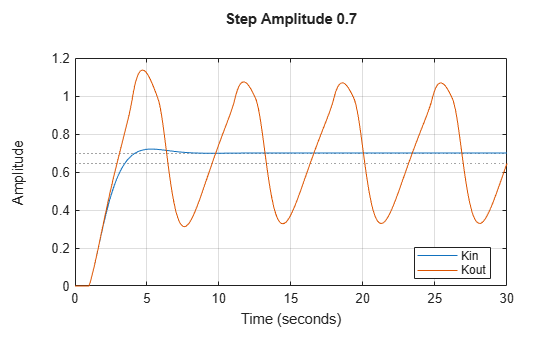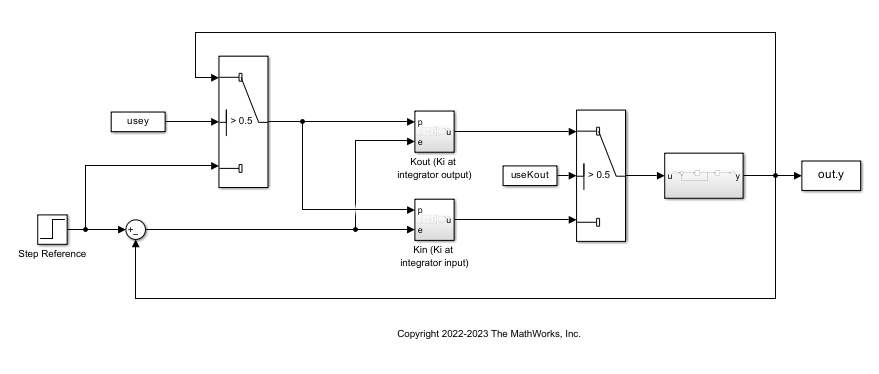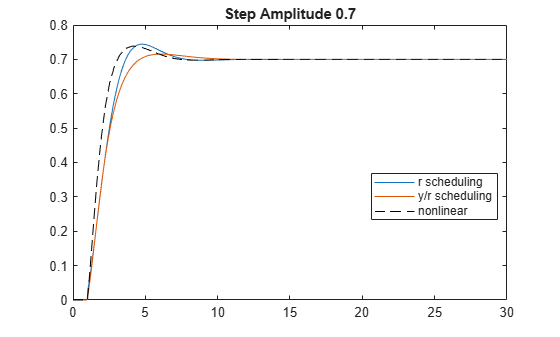# Hidden Couplings in Gain-Scheduled Control

This example illustrates the hidden couplings that can arise in gain-scheduled control depending on controller configuration. This corresponds to examples 2, 8, 9, and 11 in .

### LPV Plant

The following equations define the nonlinear plant.

`$\frac{\mathit{dx}}{\mathit{dt}}=-\mathit{x}+\mathit{u}$`

`$\mathit{y}=\mathrm{tanh}\left(\mathit{x}\right)$`

There is a collection of equilibrium points parameterized by $\mathit{p}=\mathit{y}\in \left[-1,1\right]$.

`$\left({\mathit{x}}_{0}\left(\mathit{p}\right),{\mathit{u}}_{0}\left(\mathit{p}\right),{\mathit{y}}_{0}\left(\mathit{p}\right)\right)=\left({\mathrm{tanh}}^{-1}\left(\mathit{p}\right),{\mathrm{tanh}}^{-1}\left(\mathit{p}\right),\mathit{p}\right)$`

Linearization around these equilibrium points yields the LPV model.

`$\stackrel{˙}{\mathit{x}}=-\left(\mathit{x}-{\mathit{x}}_{0}\left(\mathit{p}\right)\right)+\left(\mathit{u}-{\mathit{u}}_{0}\left(\mathit{p}\right)\right)$`

`$\mathit{y}={\mathit{y}}_{0}\left(\mathit{p}\right)+\left(1-{\mathit{p}}^{2}\right)\left(\mathit{x}-{\mathit{x}}_{0}\left(\mathit{p}\right)\right)$`

Use `lpvss` to construct this LPV plant. The data function is defined in the file `lpvHCModel.m`. Since ${\mathrm{tanh}}^{-1}\left(\mathit{p}\right)$ is infinite for $|\mathit{p}|=1$, clip $\mathit{p}$ to the range [–0.99,0.99] to stay away from singularities.

```pmax = 0.99; G = lpvss('p',@(t,p) lpvHCModel(t,p,pmax),'StateName','x');```

### Gain-Scheduled PI Controller

The gain-scheduled Proportional-Integral (PI) controller $\mathit{K}\left(\mathit{p}\right)$ is designed to achieve a closed loop damping ratio ${\zeta }_{\mathit{d}}=2}{3}$ and natural frequency ${\omega }_{\mathit{d}}=1$ at each point in the domain. You can construct this controller in two different forms.

• Controller ${\mathit{K}}_{\mathrm{in}}$, with the integral gain at the integrator input:

`${\stackrel{˙}{\mathit{x}}}_{\mathit{c}}={\mathit{K}}_{\mathit{i}}\left(\mathit{p}\right)\mathit{e}$`

`$\mathit{u}={\mathit{x}}_{\mathit{c}}+{\mathit{K}}_{\mathit{p}}\left(\mathit{p}\right)\mathit{e}$`

• Controller ${\mathit{K}}_{\mathrm{out}}$, with the integral gain at the integrator output:

`${\stackrel{˙}{\mathit{x}}}_{\mathit{c}}=\mathit{e}$`

`$\mathit{u}={\mathit{K}}_{\mathit{i}}\left(\mathit{p}\right){\mathit{x}}_{\mathit{c}}+{\mathit{K}}_{\mathit{p}}\left(\mathit{p}\right)\mathit{e}$`

These forms are equivalent when $\mathit{p}$ is constant. However, the two forms have different input/output responses when the parameter varies in time. This example compares these two options in terms of closed-loop performance.

This example provides the code for these two controllers in the files `hcKinFCN.m` and `hcKoutFCN.m`. Use `lpvss` to construct the two controllers.

```Kin = lpvss('p',@(t,p) hcKinFCN(t,p,pmax)); Kout = lpvss('p',@(t,p) hcKoutFCN(t,p,pmax));```

Use `feedback` to construct LPV models of the closed-loop systems for each controller.

```CLin = feedback(G*Kin,1); CLout = feedback(G*Kout,1);```

### LTI Analysis

Evaluate this closed-loop response for several fixed values of $\mathit{p}$.

Sample the LPV system for five values of $\mathit{p}$.

```Nvals = 5; pvals = linspace(-0.9,0.9,Nvals); CLinvals = sample(CLin,[],pvals); CLoutvals = sample(CLout,[],pvals);```

Plot the Bode response for fixed values of $\mathit{p}$.

`bode(CLinvals,'b',CLoutvals,'r--',{1e-2,1e1});`The location of the integral gain does not affect the controller when $\mathit{p}$ is constant. Hence, the two feedback loops have identical responses.

### LPV Simulation

Compute the step response of the closed-loop LPV models for the two step amplitudes `rstep` = `0.3` and `rstep` = `0.7`. This approximates the tracking performance of each gain-scheduled controller against the nonlinear plant. Both controllers use $\mathit{p}=\mathit{y}$ as the scheduling variable.

The feedback loop is locally unstable for $p>0.725$ with `Kout` while it remains locally stable for all for $p<1$ with `Kin` . Try reproducing these results with an LPV simulation.

```Tf = 30; tsim = linspace(0,Tf,1000); xinit = [0;0]; rstep = 0.3; Config = RespConfig('Amplitude',rstep,'Delay',1,'InitialState',xinit); pFcn = @(t,x,u) tanh(x(1)); step(CLin,CLout,tsim,pFcn,Config), grid title('Step Amplitude 0.3') legend('Kin','Kout','location','Best')``````rstep = 0.7; Config = RespConfig('Amplitude',rstep,'Delay',1,'InitialState',xinit); step(CLin,CLout,tsim,pFcn,Config), grid title('Step Amplitude 0.7') legend('Kin','Kout','location','Best')```The LPV simulation confirms that the feedback loop remains stable with `Kin` (integral gain at the integrator input) but exhibits a limit cycle with `Kout` for large setpoint changes. This cycle is only an approximation of the true nonlinear behavior since the output $\mathit{y}$ should never exceed 1. The LPV plant model is locally linear and cannot capture the saturating behavior of the function $\mathrm{tanh}$.

To further assess the fidelity of the LPV model, compare with a nonlinear simulation using the true nonlinear plant.

Open the Simulink® model of the closed-loop system.

```mdl = 'HiddenCouplings'; open_system(mdl)```Simulate the response with `Ki` at integrator output.

```usey = 1; useKout = 1; simout = sim('HiddenCouplings',Tf);```

Compare the LPV and nonlinear responses with the controller `Kout`.

```y = step(CLout,tsim,pFcn,Config); plot(tsim,y,simout.tout,simout.y,'--') title('Limit cycle detection for Kout') legend('LPV','Nonlinear','location','Best')```As expected, results don't match exactly due to the strong nonlinearity of $\mathrm{tanh}$. Still, the LPV simulation correctly predicts the limit cycle with approximately the right period.

### Scheduling on Reference Signal

Scheduling on $\mathit{y}=\mathrm{tanh}\left(\mathit{x}\right)$ creates an additional feedback loop between the plant and controller via the scheduling function. In other words, the closed-loop model simulated by `step` is quasi-LPV and the state-dependent scheduling causes instability. Since the output $\mathit{y}$ is meant to track the reference signal $\mathit{r}$, an alternative strategy is to schedule the controller on $\mathit{r}$, which is a truly exogenous signal. You can easily compare the two strategies by replace the parameter trajectory $\mathit{p}=\mathrm{tanh}\left(\mathit{x}\right)$ with $\mathit{p}=\mathit{r}$ in the `step` command.

```rstep = 0.3; Config = RespConfig('Amplitude',rstep,'Delay',1,'InitialState',xinit); r = rstep*(tsim>=1); step(CLin,CLout,'r--',tsim,r,Config), grid title('Scheduling on r, Step Amplitude 0.3') legend('Kin','Kout','location','Best')```Simulate with a larger step amplitude.

```rstep = 0.7; Config = RespConfig('Amplitude',rstep,'Delay',1,'InitialState',xinit); step(CLin,CLout,'r--',tsim,r,Config), grid title('Scheduling on r, Step Amplitude 0.7') legend('Kin','Kout','location','Best')```Both controllers now remain stable and have identical performance. This is because $\mathit{p}$ is piecewise constant, which is close enough to the LTI case when both controllers are interchangeable. Save the results with `Kout` for comparison with the hybrid scheme discussed next.

`y = step(CLout,tsim,r,Config);`

### Hybrid Approach

You can also use a hybrid approach where the LPV plant depends on $\mathit{p}=\mathit{y}$ and the PI controller is scheduled in $\mathit{r}$. To try this strategy, construct the LPV controller with a different parameter called `pc`:

```Kout = lpvss('pc',@(t,p) hcKoutFCN(t,p,pmax)); CLout = feedback(G*Kout,1);```

The closed-loop model now depends on two parameters `p` and `pc`. Set `p` to $\mathit{y}$ and `pc` to $\mathit{r}$ and simulate the response.

```pFcn = @(t,x,u) [tanh(x(1)) ; interp1(tsim,r,t)]; yh = step(CLout,tsim,pFcn,Config);```

Finally, run a nonlinear simulation with the true plant and a PI controller scheduled on $\mathit{r}$ with ${\mathit{K}}_{\mathit{i}}$ at the integrator output.

```usey = 0; useKout = 1; simout = sim('HiddenCouplings',Tf);```

Compare the $\mathit{r}$-scheduling and hybrid scheduling schemes with the true nonlinear response.

```plot(tsim,y,tsim,yh,simout.tout,simout.y,'k--') title('Step Amplitude 0.7') legend('r scheduling','y/r scheduling','nonlinear','location','Best')```Here scheduling both the plant and controller on $\mathit{r}$ yields a better approximation of the nonlinear response.

### Plant and Controller Data Functions

`type lpvHCModel`
```function [A,B,C,D,E,dx0,x0,u0,y0,Delays] = lpvHCModel(~,p,pmax) % Plant model p = max(-pmax,min(p,pmax)); tau = atanh(p); A = -1; B = 1; C = 1-p^2; D = 0; E = []; dx0 = []; x0 = tau; u0 = tau; y0 = p; Delays = []; ```
`type hcKinFCN`
```function [A,B,C,D,E,dx0,x0,u0,y0,Delays] = hcKinFCN(~,p,pmax) % PI Gains if abs(p)< pmax Kp = 1/3/(1-p^2); Ki = 1/(1-p^2); else % Protect against |p|>=1 Kp = 1/3/(1-pmax^2); Ki = 1/(1-pmax^2); end % State-space and offset data: Ki at the input A = 0; B = Ki; C = 1; D = Kp; E = []; dx0 = []; x0 = []; u0 = []; y0 = []; Delays = []; ```
`type hcKoutFCN`
```function [A,B,C,D,E,dx0,x0,u0,y0,Delays] = hcKoutFCN(~,p,pmax) % PI Gains if abs(p)< pmax Kp = 1/3/(1-p^2); Ki = 1/(1-p^2); else % Protect against |p|>=1 Kp = 1/3/(1-pmax^2); Ki = 1/(1-pmax^2); end % State-space and offset data: Ki at the output A = 0; B = 1; C = Ki; D = Kp; E = []; dx0 = []; x0 = []; u0 = []; y0 = []; Delays = []; ```

### References

1. Rugh, Wilson J., and Jeff S. Shamma. “Research on Gain Scheduling.” Automatica 36, no. 10 (October 2000): 1401–25. https://doi.org/10.1016/S0005-1098(00)00058-3.